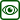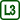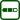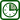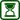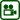## Levitating Ring – Lenz's Law Demonstration

### Experiment number : 2100

• #### Goal of experiment

The experiment shows one of the possible demonstrations of Lenz’s law.

• #### Theory

If a conductor is placed in a magnetic field, voltage is induced in the conductor. The magnitude of this voltage is given by Faraday's law of electromagnetic induction:

$U_i=-\frac{\Delta\Phi}{\Delta t},\tag{1}$

where ΔΦ is change in magnetic induction flux over time t.

If the conductor is closed, it carries an induced current. The direction of this induced current is described by Lenz’s law:

“Induced current in a closed circuit has such a direction that by its magnetic field it counteracts the change in magnetic induction flow that has caused it.”

In our case, the variable magnetic field will be generated by the coil connected to the alternating current. A lightweight aluminium ring placed on the coil core (see Fig. 1 on the left) will act as the closed conductor. Since the ring is closed, induced current will flow through it. Its direction will be, according to Lenz’s law, such that the magnetic field that surrounds the ring will be acting against the change of the magnetic flux that caused it – therefore, the magnetic field of the ring will be repelled from the magnetic field of the coil. The ring is relatively light and made of a conductive material, the induced current will therefore be so large that the ring will levitate due to the repulsive magnetic force.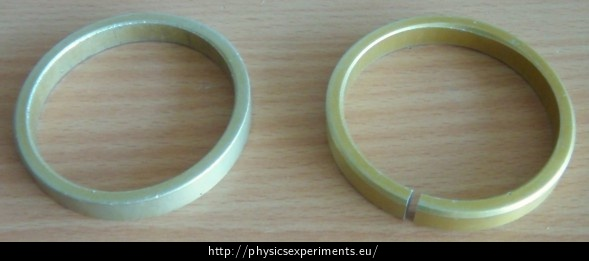A different scenario occurs when we use a cut ring (in Figure 1 on the right): voltage will be induced in the ring, however no current will be induced since the ring is not closed. Therefore, no magnetic field will be generated around it and the ring will not levitate.

• #### Tools

• coil with 300 turns
• laminated U core
• long I core
• AC 42 V power supply
• two aluminium rings (one closed, one cut)
• connecting wires

• #### Procedure

1. Put the coil on one side of the U core. Use the I core to extend this side.
2. Connect the coil to an AC power supply of approximately 40 V.
3. Place the aluminium ring on the extended core, turn on the power supply and observe that the ring rises and floats a few centimetres above the coil.

After performing and explaining the experiment, it is appropriate to propose a problem experiment to the students – instead of the closed ring, place a cut ring on the core in such a way, that the students cannot see the cut part. Let them explain this problem (see Pedagogical notes).

• #### Sample result

The sample result can be seen in the video:

• #### Problem experiment

The following video shows an experiment with another ring, which does not levitate. The video can be used as a problem task for students (see Pedagogical notes).

• #### Pedagogical notes

The experiment with a cut ring is suitable as a problem task – the teacher will show the students the experiment so that the cut is not visible, the students’ task is to suggest hypotheses why the ring does not levitate. If possible, it is advisable for the teacher to carry out the experiment with the same layout and in the same order as the experiment with the uncut ring to make sure that the problem is not, for example, in broken conductors. The students should realize that the condition for magnetic field generation around the ring is that current must flow through it; induced voltage will not make the ring to levitate.